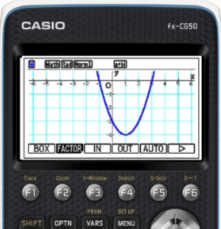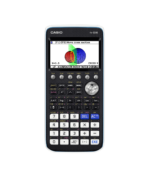Resources Archive - Casio Calculators

# Resources

Welcome to the Casio Resources Centre where you can find, view, save and download over 200 resources, created by teachers for teachers. Below you will find our most popular collections. Simply use the pre-defined collection buttons or filter facility to source the resources you need. Create your own account so that you can save and build your own resources list.

Filter

### Probability Distributions22 Resources

View AllHow to Video
fx-CG50 Further Maths
Quick Skill: Poisson and Geometric Distributions – Finding X-Values

How to calculate X-values and critical values when using the Distribution app. Y...How to Video
fx-CG50 Further Maths
Quick Skill: Geometric and Poisson Distributions – Probabilities

How to calculate Geometric and Poisson probabilities. You will need to know how ...How to Video
fx-CG50 A-Level IB
Quick Skill: Normal Distribution – Extracting X-Values

How to extract X-values calculated using the Distribution app. You will need to ...How to Video
fx-CG50 A-Level IB
Quick Skill: Probability Distributions – Using Probability Results

How to extract probabilities calculated using the Distribution app. You will nee...

### Calculus13 Resources

View AllHow to Video
fx-CG50 A-Level IB
Quick Skill: Calculations Using Function Notation

How to use function notation to perform operations in the Run-Matrix app. You wi...How to Video
fx-CG50 A-Level IB
Calculus in Mechanics – Derivatives

How to analyse the position equation of a particle to find average, instant and ...How to Video
fx-CG50 A-Level IB
Calculus in Mechanics – Derivative Functions and Integration

How to plot and analyse the relationship between the derivative functions of the...How to Video
fx-CG50 A-Level
Tangents and Normals – Parametric Functions

How to sketch the tangent and normal to a curve in parametric form. Includes fin...

### Functions50 Resources

View AllHow to Video
fx-CG50 A-Level GCSE IB
Quick Skill: Calculations Using Coordinate Values

How to extract coordinate values from points of interest in Graph to perform ope...How to Video
fx-CG50 A-Level GCSE IB
Quick Skill: Graphing – Storing and Recalling Functions

How to use Graph Memory to store functions and settings for later recall. You wi...How to Video
fx-CG50 A-Level GCSE IB
Quick Skill: Table – Extracting Values

How to extract either a single column or a complete set of table values. You wil...How to Video
fx-CG50 A-Level GCSE IB
Quick Skill: Graphing – Drawing a Graph and Table

How to draw the graph of a function and create a table of values from the graph....

### Trigonometry11 Resources

View AllHow to Video
fx-CG50 A-Level GCSE IB
Quick Skill: Calculations Using Coordinate Values

How to extract coordinate values from points of interest in Graph to perform ope...How to Video
fx-CG50 A-Level GCSE IB
Quick Skill: Graphing – Storing and Recalling Functions

How to use Graph Memory to store functions and settings for later recall. You wi...How to Video
fx-CG50 A-Level GCSE IB
Quick Skill: Table – Extracting Values

How to extract either a single column or a complete set of table values. You wil...How to Video
fx-CG50 A-Level GCSE IB
Quick Skill: Graphing – Drawing a Graph and Table

How to draw the graph of a function and create a table of values from the graph....

### Sequences and Series5 Resources

View AllHow to Video
fx-CG50 A-Level GCSE IB
Quick Skill: Sequences – Generating Terms

How to generate a list of terms of a sequence based on a function and then sum t...How to Video
fx-CG50 A-Level IB
Quick Skill: Sequences – Summation

How to use sigma summation of the terms of a sequence....How to Leaflet
fx-CG50 A-Level IB
Recursion Quick Start fx-CG50

How to create a table of sequence values on the fx-CG50. Includes plotting a rec...How to Video
fx-CG50 A-Level
Sequences – Recursion and Equations

How to iterate using ANS to generate terms in arithmetic and geometric sequences...

### Mechanics3 Resources

View AllHow to Video
fx-CG50 A-Level GCSE IB
Quick Skill: Solving Equations with more than 1 Variable

How to use Solver to solve equations with more than one variable including SUVAT...How to Video
fx-CG50 A-Level IB
Calculus in Mechanics – Derivatives

How to analyse the position equation of a particle to find average, instant and ...How to Video
fx-CG50 A-Level IB
Calculus in Mechanics – Derivative Functions and Integration

How to plot and analyse the relationship between the derivative functions of the...

### Coordinate Geometry22 Resources

View AllHow to Video
fx-CG50 A-Level
Tangents and Normals – Parametric Functions

How to sketch the tangent and normal to a curve in parametric form. Includes fin...How to Video
fx-CG50 A-Level IB
Tangents and Normals – Cartesian Functions

How to sketch the tangent and normal to a curve in Cartesian form. Includes find...How to Video
fx-CG50 A-Level
Simultaneous Equations – Solving Harder Equations Graphically

How to solve a simultaneous linear and quadratic equation in both x and y graphi...

### Number and Calculation3 Resources

View AllTeach Leaflet
fx-CG50 A-Level
Magnitude of Vector

This activity provides learner notes for finding the magnitude of a vector in tw...Teach Leaflet
fx-CG50 A-Level GCSE IB
Negative and Fractional Indices

This guided investigation establishes the rules for negative and fractional powe...Teach Leaflet
fx-CG50 A-Level GCSE IB
Simplifying Surds

A matching card exercise with a mixture of expressions which simplify to one of ...

### Algebra and Proof4 Resources

View AllTeach Leaflet
fx-CG50 A-Level IB
Factor Theorem and Cubic Graphs

This activity consists of a set of 10 cards with cubic expressions and a range o...Teach Leaflet
fx-CG50 A-Level GCSE IB
Proof

An investigation that takes a straightforward proof by counter-example and gener...

### Equations and Inequalities8 Resources

View AllHow to Video
fx-CG50 A-Level GCSE IB
Quick Skill: Solving Equations with more than 1 Variable

How to use Solver to solve equations with more than one variable including SUVAT...How to Video
fx-CG50 A-Level GCSE IB
Quick Skill: Solving Linear Equations

How to solve a linear equation using the numerical SolveN function....Teach Leaflet
fx-CG50 A-Level
The Newton-Raphson Method

This resource guides learners to see graphically that the intersection with the ...Teach Leaflet
fx-CG50 A-Level
Solve Equations Using Fixed Point Iteration

This resource is an activity for solving equations numerically including the use...

### Statistics9 Resources

View AllTeach Calculator Files
fx-CG50 A-Level
Large Data Set Pearson Pack

This resource establishes principles for working with the Pearson Large Data Set...Teach
fx-CG50 A-Level
Large Data Set OCR Pack

This resource establishes principles for working with the OCR Large Data Set. A ...Teach
fx-CG50 A-Level
Large Data Set MEI Pack

This resource establishes principles for working with the MEI Large Data Set. A ...Teach
fx-CG50 A-Level
Large Data Set AQA Pack

This resource establishes principles for working with the AQA Large Data Set. A ...